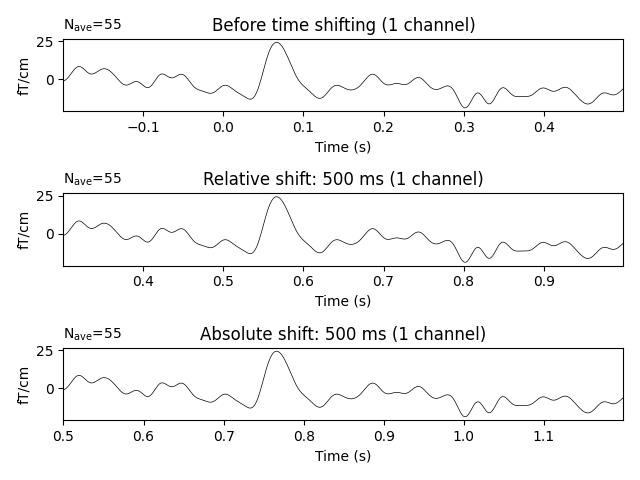# Shifting time-scale in evoked data¶Out:

Reading /home/circleci/mne_data/MNE-sample-data/MEG/sample/sample_audvis-ave.fif ...
Read a total of 4 projection items:
PCA-v1 (1 x 102) active
PCA-v2 (1 x 102) active
PCA-v3 (1 x 102) active
Average EEG reference (1 x 60) active
Found the data of interest:
t =    -199.80 ...     499.49 ms (Left Auditory)
0 CTF compensation matrices available
nave = 55 - aspect type = 100
Projections have already been applied. Setting proj attribute to True.
Applying baseline correction (mode: mean)
Need more than one channel to make topography for grad. Disabling interactivity.
Need more than one channel to make topography for grad. Disabling interactivity.
Need more than one channel to make topography for grad. Disabling interactivity.


# Author: Mainak Jas <mainak@neuro.hut.fi>
#

import matplotlib.pyplot as plt
import mne
from mne.viz import tight_layout
from mne.datasets import sample

print(__doc__)

data_path = sample.data_path()

fname = data_path + '/MEG/sample/sample_audvis-ave.fif'

condition = 'Left Auditory'
evoked = mne.read_evokeds(fname, condition=condition, baseline=(None, 0),
proj=True)

ch_names = evoked.info['ch_names']
picks = mne.pick_channels(ch_names=ch_names, include=["MEG 2332"])

# Create subplots
f, (ax1, ax2, ax3) = plt.subplots(3)
evoked.plot(exclude=[], picks=picks, axes=ax1,

# Apply relative time-shift of 500 ms
evoked.shift_time(0.5, relative=True)

evoked.plot(exclude=[], picks=picks, axes=ax2,

# Apply absolute time-shift of 500 ms
evoked.shift_time(0.5, relative=False)

evoked.plot(exclude=[], picks=picks, axes=ax3,